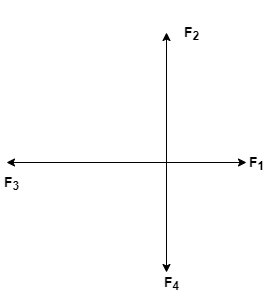# Problem: An object, which is initially at rest on a frictionless horizontal surface, is acted upon by four constant forces. F1 is 18.1 N acting due East, F2 is 37.0 N acting due North, F3 is 39.2 N acting due West, and F4 is 27.3 N actings due South. How much total work is done on the object in 3.33 seconds, if it has a mass of 18.0 kg?Which type of energy is changing for the object while the above work is being done?A) internal energyB) gravitational potential energyc) elastic potential energyD) kinetic energy

###### FREE Expert Solution

In this problem, we're going to consider Newton's second law of motion:

$\overline{){\mathbf{F}}{\mathbf{=}}{\mathbf{m}}{\mathbf{a}}}$

Work is given as:

$\overline{){\mathbf{W}}{\mathbf{=}}{\mathbf{F}}{\mathbf{·}}{\mathbf{s}}}$, where s is the distance traveled by the object.

In our case, the distance s is given, from the kinematic equations, by:

$\overline{){\mathbf{s}}{\mathbf{=}}{{\mathbf{v}}}_{{\mathbf{0}}}{\mathbf{t}}{\mathbf{+}}\frac{\mathbf{1}}{\mathbf{2}}{\mathbf{a}}{{\mathbf{t}}}^{{\mathbf{2}}}}$

The four forces can be represented in the diagram below:85% (442 ratings)###### Problem Details

An object, which is initially at rest on a frictionless horizontal surface, is acted upon by four constant forces. F1 is 18.1 N acting due East, F2 is 37.0 N acting due North, F3 is 39.2 N acting due West, and F4 is 27.3 N actings due South. How much total work is done on the object in 3.33 seconds, if it has a mass of 18.0 kg?

Which type of energy is changing for the object while the above work is being done?

A) internal energy
B) gravitational potential energy
c) elastic potential energy
D) kinetic energy